# Primary 6 SA2 Practice #3

Time elapsed:
Q1. How many letters below have both parallel and perpendicular lines?     [1 mark]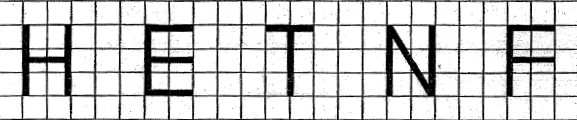Q2. 3817 cm = ____ m     [1 mark]
Q3. Zoe took a flight from 06 45 to 16 00. How long was the flight?     [1 mark]
Q4. In the figure below, ABCE is a rectangle with AB = 10 cm and BC = 4 cm. ED = 3 CM and AD = 5 cm. Find the area of the shaded triangle.     [1 mark]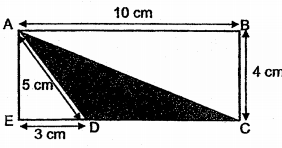Q5. Which one of the following would be the most likely radius of a wheel of a bus?     [1 mark]
Q6. Francis had 7 ℓ of juice. He drank 12 of it and gave 14 ℓ to his friend. How much juice had he left?     [2 marks]
Q7. The diagram shows semi-circles with 3 different diameters. What fraction of the figure is shaded?     [1 mark]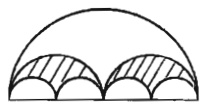Q8. Which of the following fractions is the nearest to 0.8?     [1 mark]
Q9. How many ten thousands are there in 4 710 000?     [1 mark]
Q10. ABCD is a rhombus. Which line is parallel to AB?     [1 mark]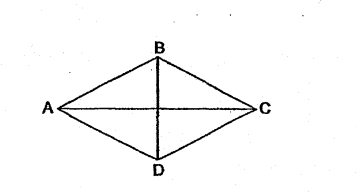Q11. Paula read 15 of the pages of a story book on Monday, 14 of the remainder of Tuesday and 12 of what remained on Wednesday. She read the final 60 pages on Thursday. How many pages were there in the story book?     [2 marks]
Q12. If a : b = 1 : 3 and b : c = 2 : 5, what is a : c?     [1 mark]
Q13. Simplify 11n - 2 - 3n x 3 + 5.     [2 marks]
Q14. 4 tens and 28 hundredths is _____.     [1 mark]
Q15. Zoe had 9 kg of flour. She used 13 of it to bake a cake. After that, she used 12 kg of the flour to bake some cookies. How much flour had she left?     [2 marks]
Q16. The figure below is made up of a quadrant and 2 identical semicircles. AC is twice of AD. What is the perimeter of the shaded figure below? (Take π = 22/7)     [2 marks]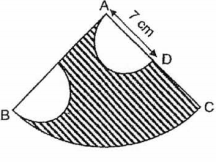Q17. Express 4.2 as a percentage.     [1 mark]
Q18. How many eighths are there in 2 34?     [1 mark]
Q19. The figure below is made up 3 of squares. Find the shaded area.     [2 marks]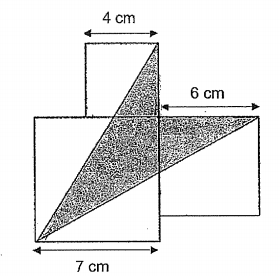Q20. The line graph below shows the number of members in a fitness club from 2014 to 2018. How many members were there in 2016?     [1 mark]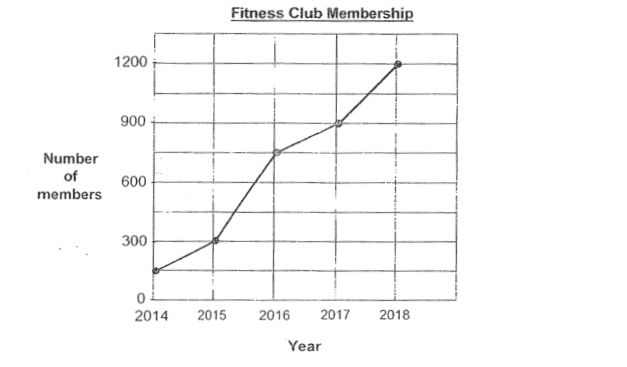Q21. Arrange the fractions, 25, 89, 17 from the biggest to the smallest.     [1 mark]
Q22. Which of the following fractions is nearest to 17?     [1 mark]
Q23. Which of the following is eight hundred and five thousand and twenty-one in figures?     [1 mark]
Q24. The diagram below shows the fuel gauge of a private airplane. If the tank has a capacity of 289ℓ, which of the following is the best estimate of the amount of fuel used?     [2 marks]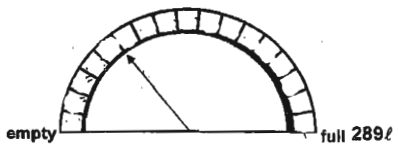Q25. Triangle ABC below is an equilateral triangle. Find the value of ∠x.     [1 mark]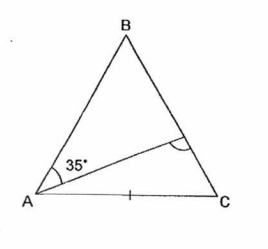Q26. In the figure below, ABC is a straight line. ∠y is 24° smaller than ∠x. Find ∠x.     [2 marks]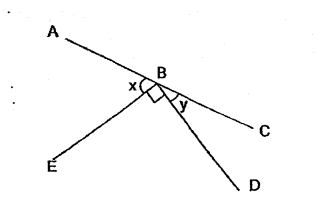Q27. Xavier paid \$900 for a laptop at a discount of 10% at an electronics shop. He signed up as a member of the shop and enjoyed an additional 10% discount on top of the discounted price. What was the total discount Xavier received for buying the laptop?     [2 marks]
Q28. Alex had some magazines. He sold 315 magazines from Monday to Friday. He sold 25 of the remaining magazines on Saturday and Sunday. The number of magazines left was 14 of what had a first. How many magazines did he have at first?     [2 marks]
Q29. In the figure below, ∠BCD = 183°, ∠ECD = 117° and ∠ACE = 45°. Find ∠BCA.     [1 mark]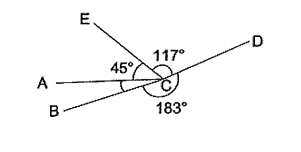Q30. How many whole numbers from 29 to 62 are divisible by 5?     [1 mark]